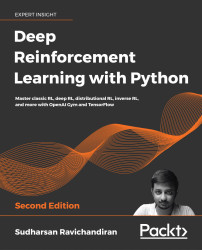•#### Deep Reinforcement Learning with Python - Second Edition#### Overview of this book

With significant enhancements in the quality and quantity of algorithms in recent years, this second edition of Hands-On Reinforcement Learning with Python has been revamped into an example-rich guide to learning state-of-the-art reinforcement learning (RL) and deep RL algorithms with TensorFlow 2 and the OpenAI Gym toolkit. In addition to exploring RL basics and foundational concepts such as Bellman equation, Markov decision processes, and dynamic programming algorithms, this second edition dives deep into the full spectrum of value-based, policy-based, and actor-critic RL methods. It explores state-of-the-art algorithms such as DQN, TRPO, PPO and ACKTR, DDPG, TD3, and SAC in depth, demystifying the underlying math and demonstrating implementations through simple code examples. The book has several new chapters dedicated to new RL techniques, including distributional RL, imitation learning, inverse RL, and meta RL. You will learn to leverage stable baselines, an improvement of OpenAI’s baseline library, to effortlessly implement popular RL algorithms. The book concludes with an overview of promising approaches such as meta-learning and imagination augmented agents in research. By the end, you will become skilled in effectively employing RL and deep RL in your real-world projects.
PrefaceFundamentals of Reinforcement LearningFree Chapter
A Guide to the Gym ToolkitThe Bellman Equation and Dynamic ProgrammingMonte Carlo MethodsUnderstanding Temporal Difference LearningCase Study – The MAB ProblemDeep Learning FoundationsA Primer on TensorFlowDeep Q Network and Its VariantsActor-Critic Methods – A2C and A3CLearning DDPG, TD3, and SACTRPO, PPO, and ACKTR MethodsDistributional Reinforcement LearningImitation Learning and Inverse RLDeep Reinforcement Learning with Stable BaselinesReinforcement Learning FrontiersOther Books You May EnjoyIndexAppendix 1 – Reinforcement Learning Algorithms# Training a cheetah bot to run using PPO

In this section, let's learn how to train the 2D cheetah bot to run using Proximal Policy Optimization (PPO). First, import the necessary libraries:

``````import gym
from stable_baselines.common.policies import MlpPolicy
from stable_baselines.common.vec_env import DummyVecEnv, VecNormalize
from stable_baselines import PPO2
``````

Create a vectorized environment using `DummyVecEnv`:

``````env = DummyVecEnv([lambda: gym.make("HalfCheetah-v2")])
``````

Normalize the state:

``````env = VecNormalize(env,norm_obs=True)
``````

Instantiate the agent:

``````agent = PPO2(MlpPolicy, env)
``````

Train the agent:

``````agent.learn(total_timesteps=250000)
``````

After training, we can see how our trained cheetah bot learned to run by rendering the environment:

``````state = env.reset()
while True:
action, _ = agent.predict(state)
next_state, reward, done, info = env.step(action)
state = next_state
env.render()
``````

Save the whole code used in this...